## Mandelbrot SetThe set obtained by the Quadratic Recurrence(1)

where points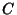for which the orbitdoes not tend to infinity are in the Set. It marks the set of points in the Complex Plane such that the corresponding Julia Set is Connected and not Computable. The Mandelbrot set was originally called a Mu Molecule by Mandelbrot.

J. Hubbard and A. Douady proved that the Mandelbrot set is Connected. Shishikura (1994) proved that the boundary of the Mandelbrot set is a Fractal with Hausdorff Dimension 2. However, it is not yet known if the Mandelbrot set is pathwise-connected. If it is pathwise-connected, then Hubbard and Douady's proof implies that the Mandelbrot set is the image of a Circle and can be constructed from a Disk by collapsing certain arcs in the interior (Douady 1986).

The Area of the set is known to lie between 1.5031 and 1.5702; it is estimated as 1.50659....

Decomposing the Complex coordinateandgives(2)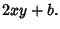(3)

In practice, the limit is approximated by(4)

Beautiful computer-generated plots can be created by coloring nonmember points depending on how quickly they diverge to. A common choice is to define an Integer called the Count to be the largestsuch that, where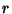is usually taken as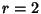, and to color points of different Count different colors. The boundary between successive Counts defines a series of Lemniscates,'' called Equipotential Curves by Peitgen and Saupe (1988),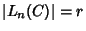which have distinctive shapes. The first few Lemniscates are(5)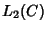(6)(7)(8)

When written in Cartesian Coordinates, the first three of these are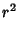(9)(10)(11)

which are a Circle, an Oval, and a Pear Curve. In fact, the second Lemniscatecan be written in terms of a new coordinate system withas(12)

which is just a Cassini Oval withand. The Lemniscates grow increasingly convoluted with higher Count and approach the Mandelbrot set as the Count tends to infinity.The kidney bean-shaped portion of the Mandelbrot set is bordered by a Cardioid with equations(13)(14)

The adjoining portion is a Circle with center at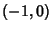and Radius. One region of the Mandelbrot set containing spiral shapes is known as Sea Horse Valley because the shape resembles the tail of a sea horse.

Generalizations of the Mandelbrot set can be constructed by replacingwith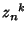or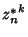, whereis a Positive Integer anddenotes the Complex Conjugate of. The following figures show the Fractals obtained for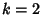, 3, and 4 (Dickau). The plots on the right havereplaced withand are sometimes called Mandelbar Sets.''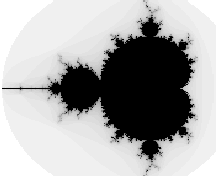See also Cactus Fractal, Fractal, Julia Set, Lemniscate (Mandelbrot Set), Mandelbar Set, Quadratic Map, Randelbrot Set, Sea Horse Valley

References

Alfeld, P. The Mandelbrot Set.'' http://www.math.utah.edu/~alfeld/math/mandelbrot/mandelbrot1.html.

Branner, B. The Mandelbrot Set.'' In Chaos and Fractals: The Mathematics Behind the Computer Graphics, Proc. Sympos. Appl. Math., Vol. 39 (Ed. R. L. Devaney and L. Keen). Providence, RI: Amer. Math. Soc., 75-105, 1989.

Dickau, R. M. Mandelbrot (and Similar) Sets.'' http://forum.swarthmore.edu/advanced/robertd/mandelbrot.html.

Douady, A. Julia Sets and the Mandelbrot Set.'' In The Beauty of Fractals: Images of Complex Dynamical Systems (Ed. H.-O. Peitgen and D. H. Richter). Berlin: Springer-Verlag, p. 161, 1986.

Eppstein, D. Area of the Mandelbrot Set.'' http://www.ics.uci.edu/~eppstein/junkyard/mand-area.html.

Fisher, Y. and Hill, J. Bounding the Area of the Mandelbrot Set.'' Submitted.

Hill, J. R. Fractals and the Grand Internet Parallel Processing Project.'' Ch. 15 in Fractal Horizons: The Future Use of Fractals. New York: St. Martin's Press, pp. 299-323, 1996.

Lauwerier, H. Fractals: Endlessly Repeated Geometric Figures. Princeton, NJ: Princeton University Press, pp. 148-151 and 179-180, 1991.

Munafo, R. Mu-Ency--The Encyclopedia of the Mandelbrot Set.'' http://home.earthlink.net/~mrob/muency.html.

Peitgen, H.-O. and Saupe, D. (Eds.). The Science of Fractal Images. New York: Springer-Verlag, pp. 178-179, 1988.

Shishikura, M. The Boundary of the Mandelbrot Set has Hausdorff Dimension Two.'' Astérisque, No. 222, 7, 389-405, 1994.